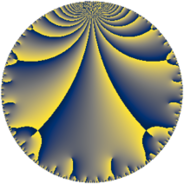# Properties

 Label 43.6.cLevel $43$ Weight $6$ Character orbit 43.c Rep. character $\chi_{43}(6,\cdot)$ Character field $\Q(\zeta_{3})$ Dimension $34$ Newform subspaces $1$ Sturm bound $22$ Trace bound $0$

# Related objects

## Defining parameters

 Level: $$N$$ $$=$$ $$43$$ Weight: $$k$$ $$=$$ $$6$$ Character orbit: $$[\chi]$$ $$=$$ 43.c (of order $$3$$ and degree $$2$$) Character conductor: $$\operatorname{cond}(\chi)$$ $$=$$ $$43$$ Character field: $$\Q(\zeta_{3})$$ Newform subspaces: $$1$$ Sturm bound: $$22$$ Trace bound: $$0$$

## Dimensions

The following table gives the dimensions of various subspaces of $$M_{6}(43, [\chi])$$.

Total New Old
Modular forms 38 38 0
Cusp forms 34 34 0
Eisenstein series 4 4 0

## Trace form

 $$34q - 14q^{2} - 14q^{3} + 454q^{4} + 71q^{5} + 15q^{6} + 225q^{7} - 936q^{8} - 1011q^{9} + O(q^{10})$$ $$34q - 14q^{2} - 14q^{3} + 454q^{4} + 71q^{5} + 15q^{6} + 225q^{7} - 936q^{8} - 1011q^{9} - 317q^{10} + 1326q^{11} - 648q^{12} + 1006q^{13} - 1272q^{14} + 683q^{15} + 3574q^{16} + 200q^{17} + 1861q^{18} + 3361q^{19} + 3825q^{20} - 1320q^{21} - 8768q^{22} + 560q^{23} - 7382q^{24} - 3232q^{25} - 3201q^{26} - 122q^{27} + 13934q^{28} + 8887q^{29} - 19449q^{30} - 6749q^{31} - 19086q^{32} + 7106q^{33} + 8423q^{34} + 31118q^{35} - 14112q^{36} - 4514q^{37} + 7072q^{38} - 5404q^{39} - 18519q^{40} - 28996q^{41} + 58118q^{42} - 14998q^{43} + 71050q^{44} - 92096q^{45} + 20052q^{46} - 10742q^{47} + 32927q^{48} + 7472q^{49} + 20362q^{50} + 20250q^{51} + 59532q^{52} - 50572q^{53} - 230084q^{54} + 38544q^{55} - 40355q^{56} - 18087q^{57} - 33436q^{58} + 112654q^{59} + 134093q^{60} - 20120q^{61} - 31491q^{62} + 188227q^{63} + 125164q^{64} - 36578q^{65} + 8803q^{66} - 73824q^{67} - 128456q^{68} + 8005q^{69} - 141610q^{70} + 142842q^{71} + 98466q^{72} - 91624q^{73} - 99720q^{74} + 298358q^{75} + 258288q^{76} + 68051q^{77} - 201116q^{78} + 99734q^{79} - 31261q^{80} - 28441q^{81} - 147772q^{82} - 47340q^{83} - 624232q^{84} - 71734q^{85} - 115526q^{86} - 215924q^{87} - 720684q^{88} + 60402q^{89} + 676108q^{90} + 164172q^{91} + 78997q^{92} - 40793q^{93} - 341874q^{94} + 123541q^{95} - 442140q^{96} + 318476q^{97} + 217473q^{98} - 134770q^{99} + O(q^{100})$$

## Decomposition of $$S_{6}^{\mathrm{new}}(43, [\chi])$$ into newform subspaces

Label Dim. $$A$$ Field CM Traces $q$-expansion
$$a_2$$ $$a_3$$ $$a_5$$ $$a_7$$
43.6.c.a $$34$$ $$6.897$$ None $$-14$$ $$-14$$ $$71$$ $$225$$